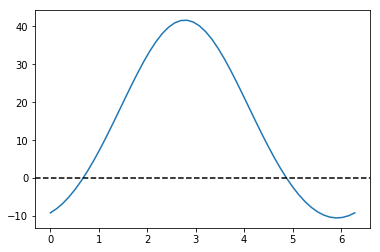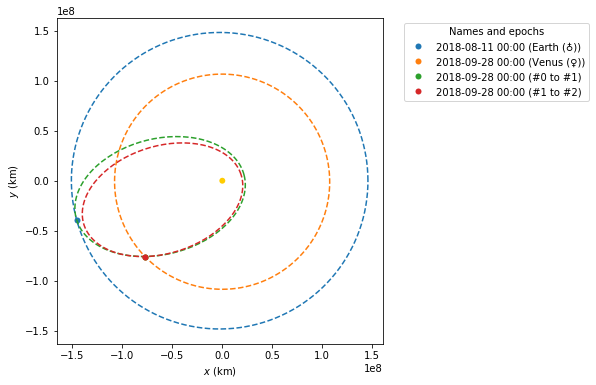# Analyzing the Parker Solar Probe flybys¶

## 1. Modulus of the exit velocity, some features of Orbit #2¶

First, using the data available in the reports, we try to compute some of the properties of orbit #2. This is not enough to completely define the trajectory, but will give us information later on in the process.

In :
from astropy import units as u

In :
T_ref = 150 * u.day
T_ref

Out:
$150 \; \mathrm{d}$
In :
from poliastro.bodies import Earth, Sun, Venus

In :
k = Sun.k
k

Out:
$1.3271244 \times 10^{20} \; \mathrm{\frac{m^{3}}{s^{2}}}$
In :
import numpy as np

In :
a_ref = np.cbrt(k * T_ref**2 / (4 * np.pi**2)).to(u.km)
a_ref.to(u.au)

Out:
$0.55249526 \; \mathrm{AU}$
In :
energy_ref = (-k / (2 * a_ref)).to(u.J / u.kg)
energy_ref

Out:
$-8.0283755 \times 10^{8} \; \mathrm{\frac{J}{kg}}$
In :
from poliastro.twobody import Orbit
from poliastro.util import norm

from astropy.time import Time

In :
flyby_1_time = Time("2018-09-28", scale="tdb")
flyby_1_time

Out:
<Time object: scale='tdb' format='iso' value=2018-09-28 00:00:00.000>
In :
r_mag_ref = norm(Orbit.from_body_ephem(Venus, epoch=flyby_1_time).r)
r_mag_ref.to(u.au)

Out:
$0.72573132 \; \mathrm{AU}$
In :
v_mag_ref = np.sqrt(2 * k / r_mag_ref - k / a_ref)
v_mag_ref.to(u.km / u.s)

Out:
$28.967364 \; \mathrm{\frac{km}{s}}$

## 2. Lambert arc between #0 and #1¶

To compute the arrival velocity to Venus at flyby #1, we have th necessary data to solve the boundary value problem

In :
d_launch = Time("2018-08-11", scale="tdb")
d_launch

Out:
<Time object: scale='tdb' format='iso' value=2018-08-11 00:00:00.000>
In :
ss0 = Orbit.from_body_ephem(Earth, d_launch)
ss1 = Orbit.from_body_ephem(Venus, epoch=flyby_1_time)

In :
tof = flyby_1_time - d_launch

In :
from poliastro import iod

In :
(v0, v1_pre), = iod.lambert(Sun.k, ss0.r, ss1.r, tof.to(u.s))

In :
v0

Out:
$[9.5993373,~11.298552,~2.9244933] \; \mathrm{\frac{km}{s}}$
In :
v1_pre

Out:
$[-16.980821,~23.307528,~9.1312908] \; \mathrm{\frac{km}{s}}$
In :
norm(v1_pre)

Out:
$30.248465 \; \mathrm{\frac{km}{s}}$

## 3. Flyby #1 around Venus¶

We compute a flyby using poliastro with the default value of the entry angle, just to discover that the results do not match what we expected.

In :
from poliastro.threebody.flybys import compute_flyby

In :
V = Orbit.from_body_ephem(Venus, epoch=flyby_1_time).v
V

Out:
$[648499.74,~2695078.4,~1171563.7] \; \mathrm{\frac{km}{d}}$
In :
h = 2548 * u.km

In :
d_flyby_1 = Venus.R + h
d_flyby_1.to(u.km)

Out:
$8599.8 \; \mathrm{km}$
In :
V_2_v_, delta_ = compute_flyby(v1_pre, V, Venus.k, d_flyby_1)

In :
norm(V_2_v_)

Out:
$27.755339 \; \mathrm{\frac{km}{s}}$

## 4. Optimization¶

Now we will try to find the value of θ that satisfies our requirements.

In :
def func(theta):
V_2_v, _ = compute_flyby(v1_pre, V, Venus.k, d_flyby_1, theta * u.rad)
ss_1 = Orbit.from_vectors(Sun, ss1.r, V_2_v, epoch=flyby_1_time)
return (ss_1.period - T_ref).to(u.day).value


There are two solutions:

In :
import matplotlib.pyplot as plt

In :
theta_range = np.linspace(0, 2 * np.pi)
plt.plot(theta_range, [func(theta) for theta in theta_range])
plt.axhline(0, color='k', linestyle="dashed")

Out:
<matplotlib.lines.Line2D at 0x18589c3a780>In :
func(0)

Out:
-9.142672330001131
In :
func(1)

Out:
7.09811543934556
In :
from scipy.optimize import brentq

In :
theta_opt_a = brentq(func, 0, 1) * u.rad
theta_opt_a.to(u.deg)

Out:
$38.598709 \; \mathrm{{}^{\circ}}$
In :
theta_opt_b = brentq(func, 4, 5) * u.rad
theta_opt_b.to(u.deg)

Out:
$279.3477 \; \mathrm{{}^{\circ}}$
In :
V_2_v_a, delta_a = compute_flyby(v1_pre, V, Venus.k, d_flyby_1, theta_opt_a)
V_2_v_b, delta_b = compute_flyby(v1_pre, V, Venus.k, d_flyby_1, theta_opt_b)

In :
norm(V_2_v_a)

Out:
$28.967364 \; \mathrm{\frac{km}{s}}$
In :
norm(V_2_v_b)

Out:
$28.967364 \; \mathrm{\frac{km}{s}}$

## 5. Exit orbit¶

And finally, we compute orbit #2 and check that the period is the expected one.

In :
ss01 = Orbit.from_vectors(Sun, ss1.r, v1_pre, epoch=flyby_1_time)
ss01

Out:
0 x 1 AU x 18.8 deg (HCRS) orbit around Sun (☉) at epoch 2018-09-28 00:00:00.000 (TDB)

The two solutions have different inclinations, so we still have to find out which is the good one. We can do this by computing the inclination over the ecliptic - however, as the original data was in the International Celestial Reference Frame (ICRF), whose fundamental plane is parallel to the Earth equator of a reference epoch, we have change the plane to the Earth ecliptic, which is what the original reports use.

In :
ss_1_a = Orbit.from_vectors(Sun, ss1.r, V_2_v_a, epoch=flyby_1_time)
ss_1_a

Out:
0 x 1 AU x 25.0 deg (HCRS) orbit around Sun (☉) at epoch 2018-09-28 00:00:00.000 (TDB)
In :
ss_1_b = Orbit.from_vectors(Sun, ss1.r, V_2_v_b, epoch=flyby_1_time)
ss_1_b

Out:
0 x 1 AU x 13.1 deg (HCRS) orbit around Sun (☉) at epoch 2018-09-28 00:00:00.000 (TDB)

Let’s define a function to do that quickly for us, using the  get_frame <https://docs.poliastro.space/en/latest/safe.html#poliastro.frames.get_frame> __ function from poliastro.frames:

In :
from astropy.coordinates import CartesianRepresentation
from poliastro.frames import Planes, get_frame

def change_plane(ss_orig, plane):
"""Changes the plane of the Orbit.

"""
ss_orig_rv = ss_orig.frame.realize_frame(
ss_orig.represent_as(CartesianRepresentation)
)

dest_frame = get_frame(ss_orig.attractor, plane, obstime=ss_orig.epoch)

ss_dest_rv = ss_orig_rv.transform_to(dest_frame)
ss_dest_rv.representation_type = CartesianRepresentation

ss_dest = Orbit.from_vectors(
ss_orig.attractor,
r=ss_dest_rv.data.xyz,
v=ss_dest_rv.data.differentials['s'].d_xyz,
epoch=ss_orig.epoch,
plane=plane,
)
return ss_dest

In :
change_plane(ss_1_a, Planes.EARTH_ECLIPTIC)

Out:
0 x 1 AU x 3.5 deg (HeliocentricEclipticJ2000) orbit around Sun (☉) at epoch 2018-09-28 00:00:00.000 (TDB)
In :
change_plane(ss_1_b, Planes.EARTH_ECLIPTIC)

Out:
0 x 1 AU x 13.1 deg (HeliocentricEclipticJ2000) orbit around Sun (☉) at epoch 2018-09-28 00:00:00.000 (TDB)

Therefore, the correct option is the first one.

In :
ss_1_a.period.to(u.day)

Out:
$150 \; \mathrm{d}$
In :
ss_1_a.a

Out:
$82652115 \; \mathrm{km}$

And, finally, we plot the solution:

In :
from poliastro.plotting import OrbitPlotter

In :
frame = OrbitPlotter()

frame.plot(ss0, label=Earth)
frame.plot(ss1, label=Venus)
frame.plot(ss01, label="#0 to #1")
frame.plot(ss_1_a, label="#1 to #2");

c:\users\dm02908\appdata\local\programs\python\python36\lib\site-packages\poliastro\twobody\orbit.py:494: UserWarning:

Frame <class 'astropy.coordinates.builtin_frames.icrs.ICRS'> does not support 'obstime', time values were not returned